May 14, 2014

# Laplace and Fourier transforms.

Let’s talk math because it’s cool.

Last year I took a course on ordinary differential equations. We were basically taught to identify different types of ordinary differential equations and use an appropriate method to solve them. Certain problems could be solved in multiple ways, but some methods are better than others.

One interesting method was the application of the Laplace transform (1).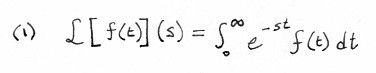The Laplace transform helps when dealing with convolutions of functions. Convolutions are functions that express the area of the overlapping region of two functions as one is translated. That’s a weird description, so here’s an animation by Brian Amberg:The Laplace transform basically takes a convolution and breaks it down into a multiplication of two functions. Working with multiplication is much easier when trying to solve differential equations, so the technique is to do all of the work within the transform and then eventually applying the inverse to get the solution you want.

Let’s go back to the equation (1). My academic mind has been in the statistics and probability realm for a bit, and so the the Laplace transform looks eerily familiar. What was the formula for moment generating functions again (2)?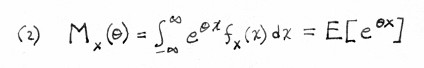Huh. That does look similar. We’ll get back to that in a bit.

That’s pretty cool. Now let’s talk about another transform: the Fourier transform (3).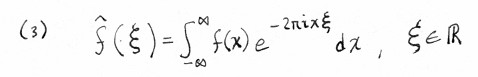The Fourier transform decomposes a function into a series of frequencies. Instead of expressing a function in terms of a time domain, we can express it in terms of a frequency domain. This is useful when dealing with sound waves, for example, which are essentially sums of sine waves. With a Fourier transform, we can “pull out” those individual sine waves. In (4) we see how we can reassemble a function in terms of its Fourier transform.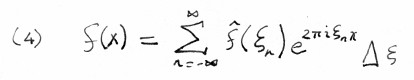Let’s go back to Laplace. Turns out that the moment generating function is the Laplace transform (two-sided – look at the limits of integration) (5). Like the Fourier transform, it also decomposes functions. However, instead of decomposing into frequencies, the Laplace transform decomposes functions into moments (which is why it’s the moment generating function).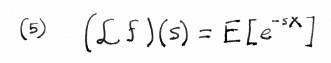And that’s what you learn when you’re bored and read Wikipedia on a phone.